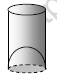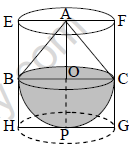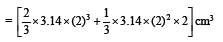# CBSE Class 10 Mathematics Surface Area And Volume Worksheet Set B

Read and download free pdf of CBSE Class 10 Mathematics Surface Area And Volume Worksheet Set B. Students and teachers of Class 10 Mathematics can get free printable Worksheets for Class 10 Mathematics Chapter 13 Surface Area and Volume in PDF format prepared as per the latest syllabus and examination pattern in your schools. Class 10 students should practice questions and answers given here for Mathematics in Class 10 which will help them to improve your knowledge of all important chapters and its topics. Students should also download free pdf of Class 10 Mathematics Worksheets prepared by school teachers as per the latest NCERT, CBSE, KVS books and syllabus issued this academic year and solve important problems with solutions on daily basis to get more score in school exams and tests

## Worksheet for Class 10 Mathematics Chapter 13 Surface Area and Volume

Class 10 Mathematics students should refer to the following printable worksheet in Pdf for Chapter 13 Surface Area and Volume in Class 10. This test paper with questions and answers for Class 10 will be very useful for exams and help you to score good marks

### Class 10 Mathematics Worksheet for Chapter 13 Surface Area and Volume

Surface Areas and Volumes

Q.- A bird bath for garden in the shape of a cylinder with a hemispherical depression at one end (see figure). The height of the cylinder is 1.45 m and its radius is 30 cm.Find the total surface area of the bird-bath.
(Take π = 22/7 )Sol. Let h be height of the cylinder and r the common radius of the cylinder and hemisphere. Then, the total surface area of the bird-bath
= CSA of cylinder + CSA of hemisphere
= 2πrh + 2πr2 = 2πr2 = 2πr(h + r)
= 2 × 22/7 × 30 (145 + 30) cm2
= 33000 cm2 = 3.3 m2

Q.- A juice seller was serving his customers using glasses as shown in figure. The inner diameter of the cylindrical glass was 5 cm,but the bottom of the glass had a hemispherical raised portion which reduced the capacity of the glass. If the height of a glass was 10 cm. Find the apparent capacity of the glass and its actual capacity. (Use π= 3.14)Sol. Since the inner diameter of the glass = 5 cm and height = 10 cm, the apparent capacity of the glass = πr2h
= 3.14 × 2.5 × 2.5 × 10 cm3 = 196.25 cm3
But the actual capacity of the glass is less by the volume of the hemisphere at the base of the glass.
i.e. it is less by 2/3 πr3

= 2/3 × 3.14 × 2.5 × 2.5 × 2.5 cm3 = 32.71 cm3
So, the actual capacity of the glass = apparent capacity of glass – volume of the hemisphere
= (196.25 – 32.71) cm3
= 163.54 cm2

Q.- A solid toy is in the form of a hemisphere surmounted by a right circular cone. The height of the cone is 2 cm and the diameter of the base is 4 cm. Determine the volume of the toy. If a right circular cylinder circumscribes the toy, find the difference of the volume of the cylinder and the toy. (Take π = 3.14)Sol. Let BPC be the hemisphere and ABC be the cone standing on the base of the hemisphere (see figure). The radius BO of the hemisphere
(as well as of the cone) = 1/2 × 4 cm = 2 cm
So, volume of the toy = 2/3 πr3 + 1/3 πr2h= 25.12 cm3
Now, let the right circular cylinder EFGH circumscribe the given solid. The radius of the base of the right circular cylinder
= HP = BO = 2 cm, and its height is
EH = AO + OP = (2 + 2) cm = 4 cm
So, the volume required
= Volume of the right circular cylinder – volume of the toy
= (3.14 × 22 × 4 – 25.12) cm3
= 25.12 cm3
= 25.12 cm3
Hence, the required difference of the two
volumes = 25.12 cm3.

Q.- A cone of height 24 cm and radius of base 6 cm is made up of modeling clay. A child reshapes it in the form of a sphere. Find the radius of the sphere.
Sol. Volume of cone = 1/3 × π × 6 × 6 × 24 cm3
If r is the radius of the sphere, then its volume is 4/3 πr3.
Since the volume of clay in the form of the cone and the sphere remains the same, we have.

4/3 × π × r3 = 1/3 × π × 6 × 6 × 24
r3 = 3 × 3 × 24 = 33 × 23
r = 3 × 2 = 6
Therefore, the radius of the sphere is 6 cm

Q.-  Selvi's house has an overhead tank in the shape of a cylinder. This is filled by pumping water from a sump (an underground tank) which is in the shape of a cuboid. The sump has dimensions 1.57 m × 1.44 m × 95 cm.The overhead tank has its radius 60 cm and height 95 cm. Find the height of the water left in the sump after the overhead tank has been completely filled with water from the sump which had been full. Compare the capacity of the tank with that of the sump. (Use π = 3.14)

Sol. The volume of water in the overhead tank equals the volume of the water removed from the shump.
Now the volume of water in the overhead
tank (cylinder) = πr2h
= 3.14 × 0.6 × 0.6 × 0.95 m3
The volume of water in the sump when full
= l × b × h = 1.57 × 1.44 × 0.95 m3
The volume of water left in the sump after filling the tank
= (1.57×1.44×0.95)–(3.14 × 0.6 × 0.6 × 0.95)] m3
= (1.57 × 0.6 × 0.6 × 0.95 × 2) m3
So, the height of the water left in the sump
= volume of water left in the sump/λ×bTherefore, the capacity of the tank is half the capacity of the sump.

Q.- A copper rod of diameter 1 cm and length 8 cm is drawn into a wire of length 18 m of uniform thickness. Find the thickness of the wire.
Sol. The volume of the rod = π ×(1/2)× 8 cm3
= 2π cm3.
The length of the new wire of the same volume = 18 m = 1800 cm
If r is the radius (in cm) of cross section of the wire, its volume = π × r2 × 1800 cm3
Therefore, π × r2 × 1800 = 2π
i.e. r2 =1/900
i.e. r =1/30
So, The diameter of the cross section i.e. the thickness of the wire is1/15 cm, i.e. 0.67 mm (approx.)

More question-

1.The lateral surface area of right circular cylinder with base radius 7cm and height 10 cm is:

2.The lateral surface area of cylinder is 176cm2 & base area 38.5cm2. Then its volume is

(A) 803cm3

(B) 380cm3

(C) 308cm3

(D) 830cm3

3.Ratio of curved surface areas of two cylinders with equal radii is:

(A) H2 : h2

(B) 2H : h

(C) H : h

(D) None

4.Two cubes of 12cm edge are joined end to end. Find the surface area of the resulting cuboid.

5.Three cubes of sides 6 cm edge are joined end to end. Find the surface area of the resulting cuboid.

6.A solid sphere of radius 6cm is melted and recast into small spherical balls each of diameter 0.6cm.Find the number of balls thus obtained.

7.How many spherical bullets can be made out of a solid cube of lead whose edge measures 55cm, each bullet being 10 cm in diameter?

8.The area of the base of a cone is 616 sq. cm. If its height is 48 cm then its total surface area is:

(A) 2681cm2 (B) 2861cm2 (C) 2816cm2 (D) None

9.Ratio of lateral surface areas of two cylinders with equal heights is .

(A) R : r (B) H : h (C) R2 : r2 (D) None

Please click the below link to access CBSE Class 10 Mathematics Surface Area And Volume Worksheet Set B

## More Study Material

### CBSE Class 10 Mathematics Chapter 13 Surface Area and Volume Worksheet

We hope students liked the above worksheet for Chapter 13 Surface Area and Volume designed as per the latest syllabus for Class 10 Mathematics released by CBSE. Students of Class 10 should download in Pdf format and practice the questions and solutions given in the above worksheet for Class 10 Mathematics on a daily basis. All the latest worksheets with answers have been developed for Mathematics by referring to the most important and regularly asked topics that the students should learn and practice to get better scores in their class tests and examinations. Studiestoday is the best portal for Class 10 students to get all the latest study material free of cost.

### Worksheet for Mathematics CBSE Class 10 Chapter 13 Surface Area and Volume

Expert teachers of studiestoday have referred to the NCERT book for Class 10 Mathematics to develop the Mathematics Class 10 worksheet. If you download the practice worksheet for one chapter daily, you will get higher and better marks in Class 10 exams this year as you will have stronger concepts. Daily questions practice of Mathematics worksheet and its study material will help students to have a stronger understanding of all concepts and also make them experts on all scoring topics. You can easily download and save all revision worksheet for Class 10 Mathematics also from www.studiestoday.com without paying anything in Pdf format. After solving the questions given in the worksheet which have been developed as per the latest course books also refer to the NCERT solutions for Class 10 Mathematics designed by our teachers

#### Chapter 13 Surface Area and Volume worksheet Mathematics CBSE Class 10

All worksheets given above for Class 10 Mathematics have been made as per the latest syllabus and books issued for the current academic year. The students of Class 10 can be rest assured that the answers have been also provided by our teachers for all worksheet of Mathematics so that you are able to solve the questions and then compare your answers with the solutions provided by us. We have also provided a lot of MCQ questions for Class 10 Mathematics in the worksheet so that you can solve questions relating to all topics given in each chapter. All study material for Class 10 Mathematics students have been given on studiestoday.

#### Chapter 13 Surface Area and Volume CBSE Class 10 Mathematics Worksheet

Regular worksheet practice helps to gain more practice in solving questions to obtain a more comprehensive understanding of Chapter 13 Surface Area and Volume concepts. Worksheets play an important role in developing an understanding of Chapter 13 Surface Area and Volume in CBSE Class 10. Students can download and save or print all the worksheets, printable assignments, and practice sheets of the above chapter in Class 10 Mathematics in Pdf format from studiestoday. You can print or read them online on your computer or mobile or any other device. After solving these you should also refer to Class 10 Mathematics MCQ Test for the same chapter.

#### Worksheet for CBSE Mathematics Class 10 Chapter 13 Surface Area and Volume

CBSE Class 10 Mathematics best textbooks have been used for writing the problems given in the above worksheet. If you have tests coming up then you should revise all concepts relating to Chapter 13 Surface Area and Volume and then take out a print of the above worksheet and attempt all problems. We have also provided a lot of other Worksheets for Class 10 Mathematics which you can use to further make yourself better in Mathematics

Where can I download latest CBSE Printable worksheets for Class 10 Mathematics Chapter 13 Surface Area and Volume

You can download the CBSE Printable worksheets for Class 10 Mathematics Chapter 13 Surface Area and Volume for latest session from StudiesToday.com

Can I download the Printable worksheets of Chapter 13 Surface Area and Volume Class 10 Mathematics in Pdf

Yes, you can click on the links above and download Printable worksheets in PDFs for Chapter 13 Surface Area and Volume Class 10 for Mathematics

Are the Class 10 Mathematics Chapter 13 Surface Area and Volume Printable worksheets available for the latest session

Yes, the Printable worksheets issued for Class 10 Mathematics Chapter 13 Surface Area and Volume have been made available here for latest academic session

How can I download the Class 10 Mathematics Chapter 13 Surface Area and Volume Printable worksheets

You can easily access the links above and download the Class 10 Printable worksheets Mathematics Chapter 13 Surface Area and Volume for each chapter

Is there any charge for the Printable worksheets for Class 10 Mathematics Chapter 13 Surface Area and Volume

There is no charge for the Printable worksheets for Class 10 CBSE Mathematics Chapter 13 Surface Area and Volume you can download everything free

How can I improve my scores by solving questions given in Printable worksheets in Class 10 Mathematics Chapter 13 Surface Area and Volume

Regular revision of practice worksheets given on studiestoday for Class 10 subject Mathematics Chapter 13 Surface Area and Volume can help you to score better marks in exams

Are there any websites that offer free test sheets for Class 10 Mathematics Chapter 13 Surface Area and Volume

Yes, studiestoday.com provides all latest NCERT Chapter 13 Surface Area and Volume Class 10 Mathematics test sheets with answers based on the latest books for the current academic session

Can test papers for Class 10 Mathematics Chapter 13 Surface Area and Volume be accessed on mobile devices

Yes, studiestoday provides worksheets in Pdf for Chapter 13 Surface Area and Volume Class 10 Mathematics in mobile-friendly format and can be accessed on smartphones and tablets.

Are worksheets for Chapter 13 Surface Area and Volume Class 10 Mathematics available in multiple languages

Yes, worksheets for Chapter 13 Surface Area and Volume Class 10 Mathematics are available in multiple languages, including English, Hindi